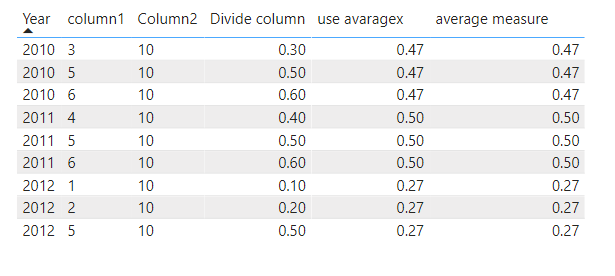cancel
Showing results for
Did you mean:

Fabric is Generally Available. Browse Fabric Presentations. Work towards your Fabric certification with the Cloud Skills Challenge.Anonymous
Not applicable

## Getting a yearly average from a measure

I have a ratio measure, and would like to take the yearly average of that measure.

Here is a screenshot of what I would like to achieve for the new average measure, Measure 2:Measure 1 is calculated from 2 columns in my dataset, using DAX: Measure 1 = DIVIDE(Table1[Column1],Table1[Column2],0)
I have tried using this formula for Measure 2:

Measure 2 = AVERAGEX(SUMMARIZE(Table1,Calendar[Year],"Average",[Measure1]),[Measure1])
But the result i get is exactly the same as Measure 1. Not sure what I'm doing wrong, am quite new to this.

Thank you for the help!
2 ACCEPTED SOLUTIONSSuper User

@Anonymous ,

Measure 2 = calculate(AvergaeX(Table[Measure1]), filter(allselected(Table),Table[Year]=max(Table[Year])))

or
Measure 2 = calculate(AvergaeX(Table[Measure1]), filter(all(Table),Table[Year]=max(Table[Year])))Community Support
Hi @Anonymous ,
According to my understanding ,you want to calculate average based on measure and different year,right?
I did it in two ways.

1.Use Average

``````Divide column =
DIVIDE ( 'average table'[column1], 'average table'[Column2], 0 )``````
``````average measure =
CALCULATE (
AVERAGE ( 'average table'[Divide column] ),
ALLEXCEPT ( 'average table', 'average table'[Year] )
)``````

2.Use Averagex

``````Measure 7 =
SUM ( 'average table'[Divide column] )``````
``````use avaragex =
AVERAGEX ( ALLEXCEPT ( 'average table', 'average table'[Year] ), [Measure 7] )``````

My visualization looks like this:Best Regards,
Eyelyn Qin
4 REPLIES 4Community Support
Hi @Anonymous ,
According to my understanding ,you want to calculate average based on measure and different year,right?
I did it in two ways.

1.Use Average

``````Divide column =
DIVIDE ( 'average table'[column1], 'average table'[Column2], 0 )``````
``````average measure =
CALCULATE (
AVERAGE ( 'average table'[Divide column] ),
ALLEXCEPT ( 'average table', 'average table'[Year] )
)``````

2.Use Averagex

``````Measure 7 =
SUM ( 'average table'[Divide column] )``````
``````use avaragex =
AVERAGEX ( ALLEXCEPT ( 'average table', 'average table'[Year] ), [Measure 7] )``````

My visualization looks like this:Best Regards,
Eyelyn QinSuper User

@Anonymous ,

Measure 2 = calculate(AvergaeX(Table[Measure1]), filter(allselected(Table),Table[Year]=max(Table[Year])))

or
Measure 2 = calculate(AvergaeX(Table[Measure1]), filter(all(Table),Table[Year]=max(Table[Year])))Anonymous
Not applicable

@amitchandak , I used your formula and changed the averagex part to this:

AVERAGEX(SUMMARIZE('Calendar','Calendar'[Date],"Avg",[Measure1]),[Avg])
and then it worked, thanks!Anonymous
Not applicable

Hi @amitchandak ,

I cant seem to use that formula. After AverageX, Table[Measure1] does not seem to be an option for the first arguement, only Table is available in the drop down list.Announcements#### Power BI Monthly Update - November 2023

Check out the November 2023 Power BI update to learn about new features.#### Fabric Community News unified experience

Read the latest Fabric Community announcements, including updates on Power BI, Synapse, Data Factory and Data Activator.#### The largest Power BI and Fabric virtual conference

130+ sessions, 130+ speakers, Product managers, MVPs, and experts. All about Power BI and Fabric. Attend online or watch the recordings.Top Solution Authors
Top Kudoed Authors
Users online (3,140)